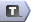# Objective Functions in AMPL

## Description

The objective function of a mathematical programme is a function of the decision variables that measures the "worth" of the decisions. This "worth" is being either maximised or minimised (although, if no objective function is defined, AMPL will use the solver to search for a feasible solution).

## Declaring an Objective Function

Objective functions are declared using either the `minimize` or `maximize` keyword and a label:

```minimize TotalCost;
```

## Defining an Objective Function

Objective functions are usually defined when they are declared, e.g.,

```minimize TotalCost: sum {i in INGREDIENTS} Cost[i] * Amount[i];
```

However, in some cases (such as when models are defined by column), the objective function is defined later

```minimize TotalWorkers;

var Work {j in SCHEDS} >= 0, integer,
obj TotalWorkers 1,
...
;
```

-- MichaelOSullivan - 02 Mar 2008

Topic revision: r1 - 2008-03-02 - MichaelOSullivanCopyright © 2008-2022 by the contributing authors. All material on this collaboration platform is the property of the contributing authors.
Ideas, requests, problems regarding TWiki? Send feedback